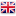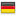# Sinergit有限公司05 十二月 2023Cr =总重量力（daN）

P =起重机的总重力（daN）

W =风力（daN）

I =水平惯性力（daN）

F =在稳定极限（daN）上两次打击所能施加的最大力

a =起重机重心与倾斜轴的距离（m）

b =距倾翻轴的负载距离（m）

c =距倾翻轴的风力距离（m）

d =水平惯性力的重心（m）

e =重心距离倾翻轴的起重机质量惯性力（m）

f =负载质量的重心与倾覆轴的距离（m）

Ms（daNm）= P * a =起重机稳定力矩

Mr（daNm）= W * c + Cr * b =起重机倾覆力矩

d =（P * e + Cr * f）/（P + Cr）

F（daN）=（女士-先生）/ d